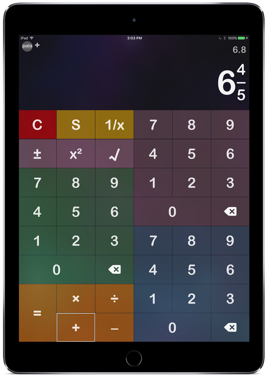Fraction Calculator  for iOS & macOS

Fractions are everywhere and the Fraction Calculator is designed to ease the task of working with fractions with its simple and intuitive calculator style interface.The Fraction Calculator feels and behaves exactly like a calculator with one exception, you're working with fractions. So now when homework (checking of course) calls for fractions, or your carpentry project needs you to add eighths and sixteenths dozens of times, or maybe you just want to be able to recalculate that dinner recipe to accommodate for five rather than two – turn to the Fraction Calculator.

Fraction Calculator allows for adding, subtracting, multiplying and dividing both positive and negative fractions, displaying the results both as a fraction and a decimal. There are also functions to allow for simplifying, reciprocals, squares and square roots.

Fraction Calculator is fully VoiceOver accessible.

Languages Supported: English.

8.0

Languages Supported: English.

Requires macOS 10.8 or laterContact